Ex 1.1

Chapter 1 Class 12 Relation and Functions
Serial order wise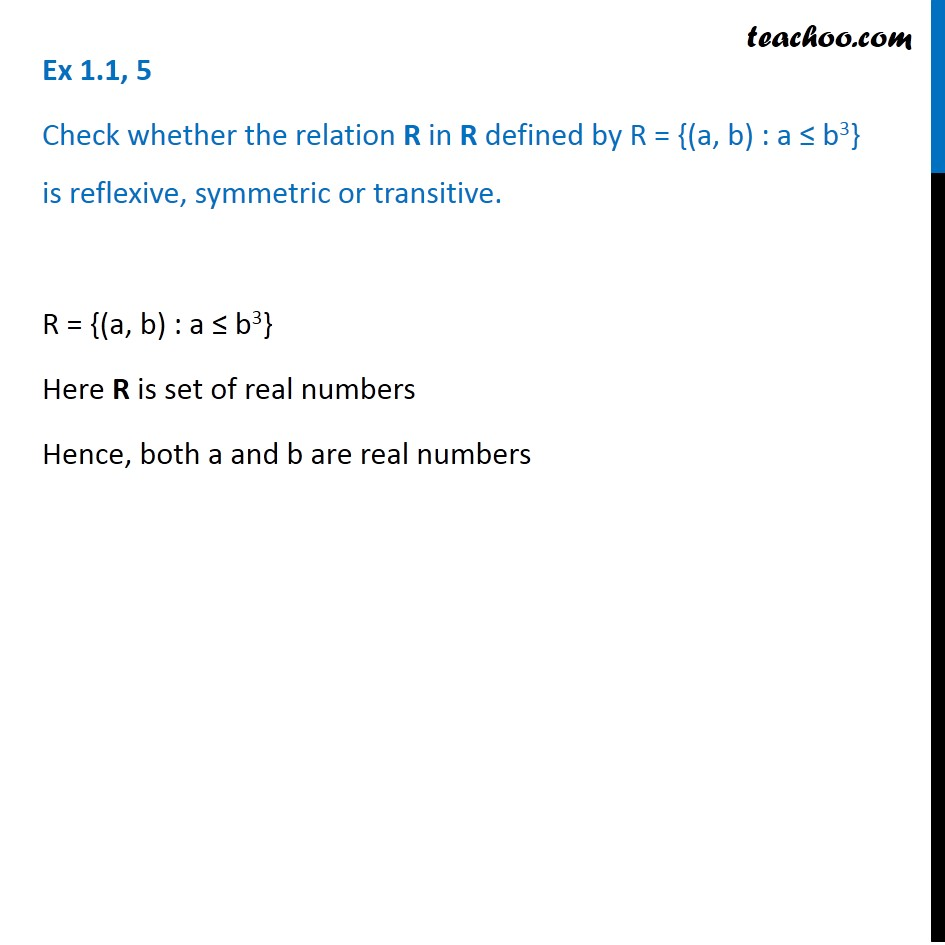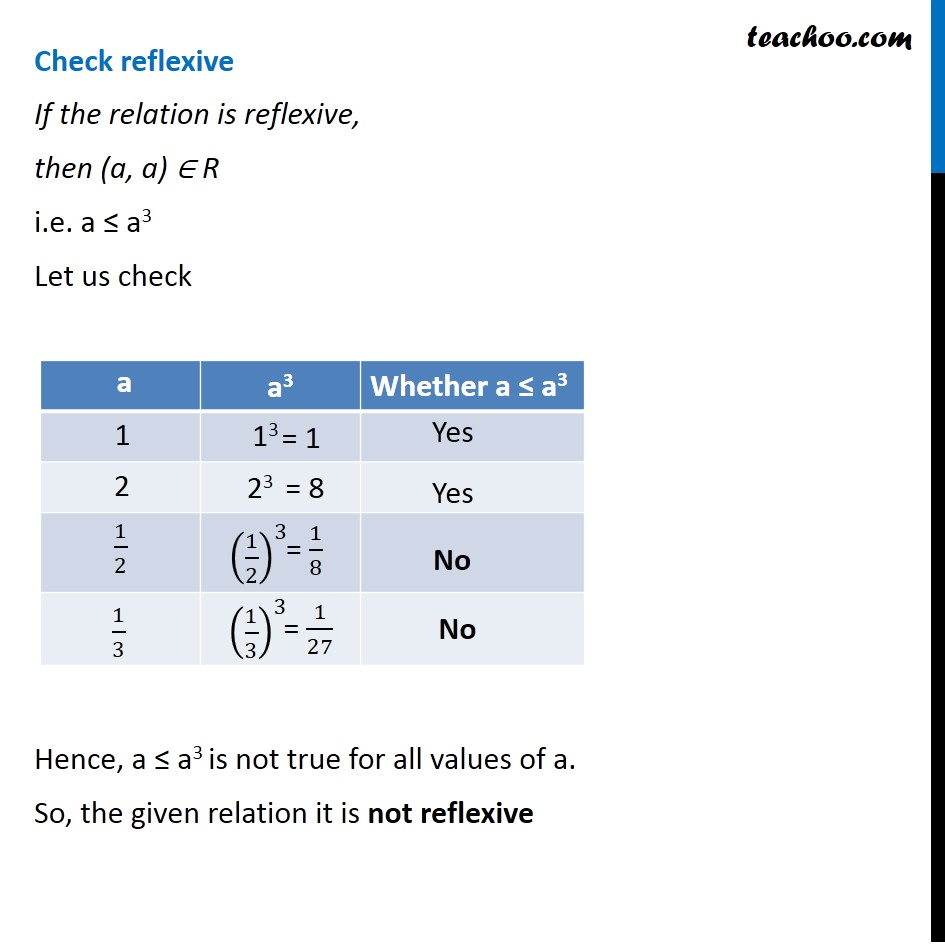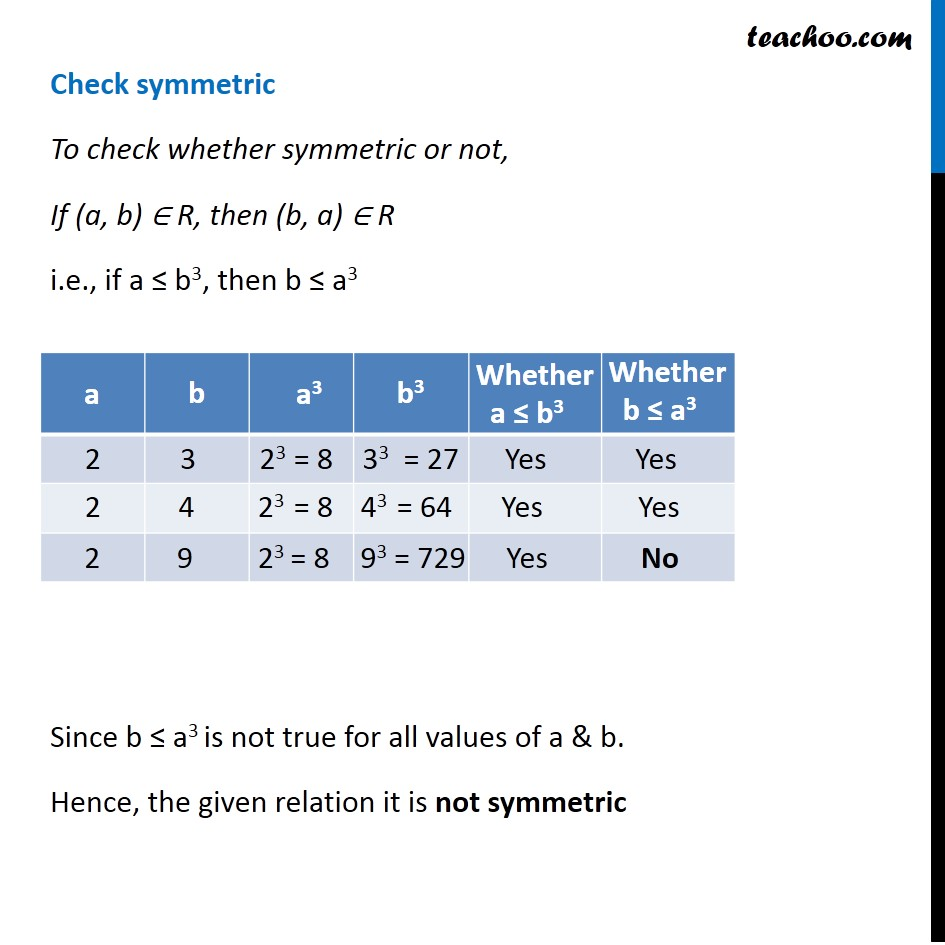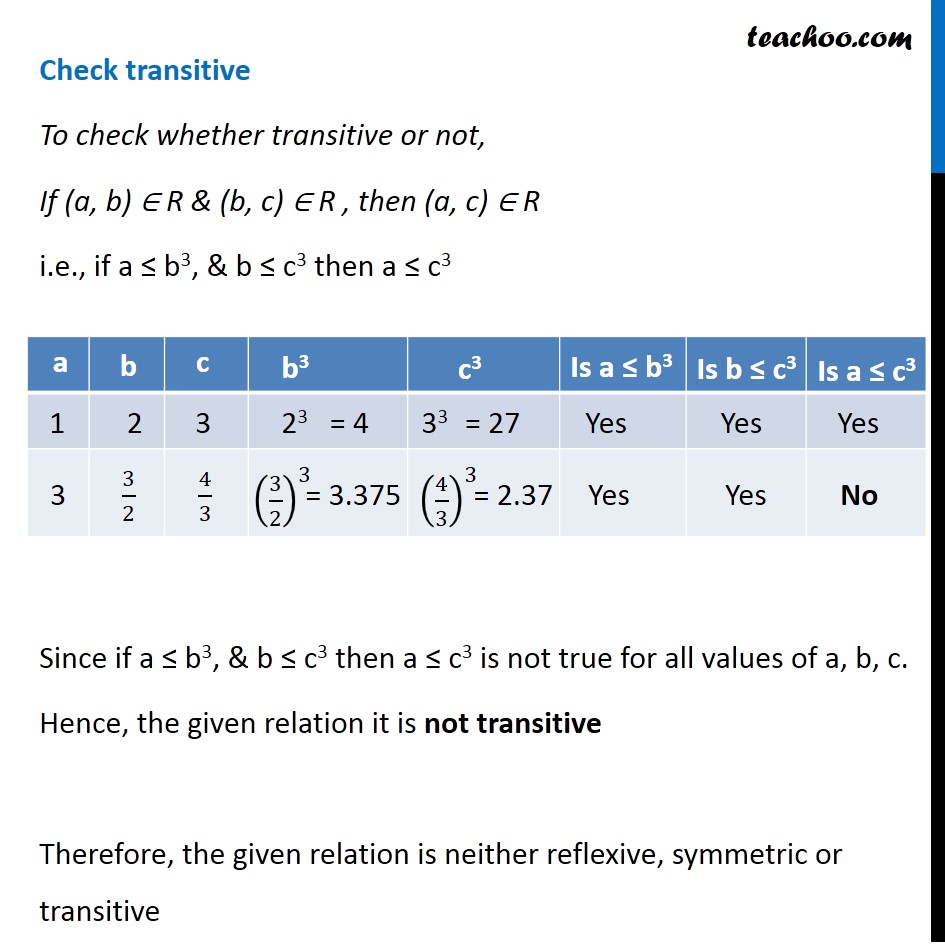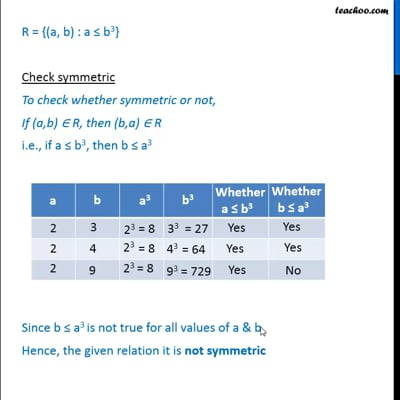This video is only available for Teachoo black users

Solve all your doubts with Teachoo Black (new monthly pack available now!)

### Transcript

Ex 1.1, 5 Check whether the relation R in R defined by R = {(a, b) : a ≤ b3} is reflexive, symmetric or transitive. R = {(a, b) : a ≤ b3} Here R is set of real numbers Hence, both a and b are real numbers Check reflexive If the relation is reflexive, then (a, a) ∈ R i.e. a ≤ a3 Let us check Hence, a ≤ a3 is not true for all values of a. So, the given relation it is not reflexive Check symmetric To check whether symmetric or not, If (a, b) ∈ R, then (b, a) ∈ R i.e., if a ≤ b3, then b ≤ a3 Since b ≤ a3 is not true for all values of a & b. Hence, the given relation it is not symmetric Check transitive To check whether transitive or not, If (a, b) ∈ R & (b, c) ∈ R , then (a, c) ∈ R i.e., if a ≤ b3, & b ≤ c3 then a ≤ c3 Since if a ≤ b3, & b ≤ c3 then a ≤ c3 is not true for all values of a, b, c. Hence, the given relation it is not transitive Therefore, the given relation is neither reflexive, symmetric or transitive